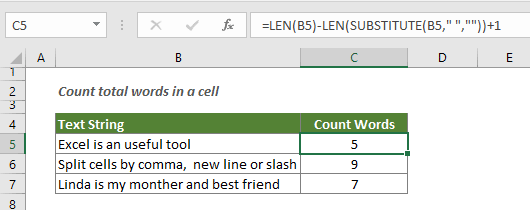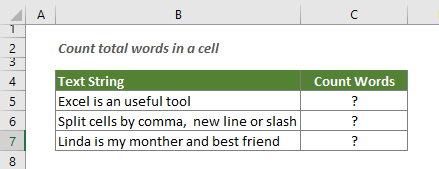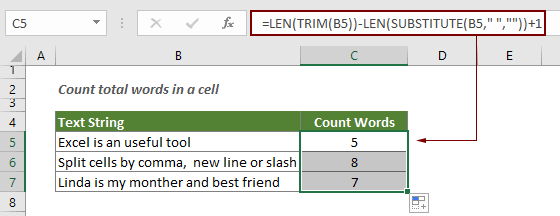## Count the number of words in a cell in Excel

This article explains how to count total words in a cell with a formula in Excel.#### How to count the number of words in a cell in Excel?

Generic formula

=LEN(TRIM(text_string))-LEN(SUBSTITUTE(text_string," ",""))+1

Argument

Text_string: The text string or sentence for which you want to count total words. It can be:
1. The text string enclosed in quotation marks;
2. Or a reference to a cell containing the text string.

How to use this formula?

Supposing you have a list with different sentences in each cell, and want to count the number of words for each cell and return the results. Please do as follows.1. Select a blank cell to output the result.

2. Enter the below formula into it and press the Enter key.

=LEN(TRIM(B5))-LEN(SUBSTITUTE(B5," ",""))+1

3. Select the first result cell, drag the Fill Handle to apply the formula to other cells. See screenshot:How this formula works?

=LEN(TRIM(B5))-LEN(SUBSTITUTE(B5," ",""))+1

• 1. LEN(SUBSTITUTE(B5," ","")): First, the SUBSTITUTE function removes all spaces from the specific cell (all spaces in the cell will be replaced with empty text string). After that, the LEN function calculates the length of the text string without spaces. Here the result is 19.
• 2. LEN(TRIM(B5)): If there are extra spaces in B5, the TRIM function will remove them and only keep a single space between words. And then the LEN function calculates the length of the text string in B5 with spaces and return 23.
• 3. LEN(TRIM(B5))-LEN(SUBSTITUTE(B5," ",""))+1: Now the length of the string with spaces (23) subtracts the string length without spaces (19), and as the number of words is equal to the number of spaces + 1, we need to add 1 to the final word count. Here the total words are 5.

#### Related functions

Excel LEN function
The LEN function returns the number of characters in a text string.

Excel SUBSTITUTE function
The SUBSTITUTE function replaces text or characters within a text string with another text or characters.

Excel TRIM function
The TRIM function removes all extra spaces from text string and only keeps single spaces between words.

#### Related formulas

Count occurrences of specific characters in an Excel cell
This tutorial introduces how to apply formulas based on the LEN and SUSTITUTE functions to count the number of times a specific character appears in an Excel cell.

Count specific characters in a range of cells
This tutorial introduces some formulas to count the number of times a specific character appears in a range in Excel.

Count specific words in a cell in Excel

Count specific words in a range in Excel
This article explains a formula to count the number of times a specific word appears in a range of cells in Excel.

Count number of characters in a cell in Excel
The total characters not only includes all letters, but also all spaces, punctuation marks and symbols in the cell. Follow this tutorial to easily count the total characters in a cell with the LEN function in Excel.

Count number of characters in a range in Excel
This article explains a formula to count total characters that appear in a range of cells in Excel.

### The Best Office Productivity Tools

#### Kutools for Excel - Helps You To Stand Out From Crowd

 Popular Features: Find, Highlight or Identify Duplicates  |  Delete Blank Rows  |  Combine Columns or Cells without Losing Data  |  Round without Formula ... Super VLookup: Multiple Criteria  |  Multiple Value  |  Across Multi-Sheets  |  Fuzzy Lookup... Adv. Drop-down List: Easy Drop Down List  |  Dependent Drop Down List  |  Multi-select Drop Down List... Column Manager: Add a Specific Number of Columns  |  Move Columns  |  Toggle Visibility Status of Hidden Columns  |  Compare Columns to Select Same & Different Cells ... Featured Features: Grid Focus  |  Design View  |  Big Formula Bar  |  Workbook & Sheet Manager | Resource Library (Auto Text)  |  Date Picker  |  Combine Worksheets  |  Encrypt/Decrypt Cells  |  Send Emails by List  |  Super Filter  |  Special Filter (filter bold/italic/strikethrough...) ... Top 15 Toolsets:  12 Text Tools (Add Text, Remove Characters ...)  |  50+ Chart Types (Gantt Chart ...)  |  40+ Practical Formulas (Calculate age based on birthday ...)  |  19 Insertion Tools (Insert QR Code, Insert Picture from Path ...)  |  12 Conversion Tools (Numbers to Words, Currency Conversion ...)  |  7 Merge & Split Tools (Advanced Combine Rows, Split Excel Cells ...)  |  ... and more

Kutools for Excel Boasts Over 300 Features, Ensuring That What You Need is Just A Click Away...#### Office Tab - Enable Tabbed Reading and Editing in Microsoft Office (include Excel)

• One second to switch between dozens of open documents!
• Reduce hundreds of mouse clicks for you every day, say goodbye to mouse hand.
• Increases your productivity by 50% when viewing and editing multiple documents.
• Brings Efficient Tabs to Office (include Excel), Just Like Chrome, Edge and Firefox.